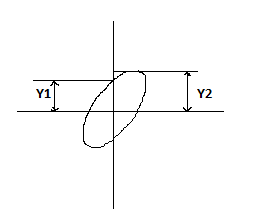# Oscilloscope - MCQs with answers

## Oscilloscope - MCQs with answers

Q1. CRO gives the visual representation of time varying signals. The display of the signal is

A. One dimensional
B. Two dimensional
C. Three dimensional
D. Four dimensional

Q2. Principally CRO is a

A. Ammeter
B. Voltmeter
C. Wattmeter
D. Watt-hour meter

Q3. The sweep generator of a CRO is used to produce

A. Sinusoidal voltage for the horizontal deflection of electron beam

B. Saw tooth voltage for the vertical deflection of electron beam

C. Sinusoidal voltage for the vertical deflection of electron beam

D. Saw tooth voltage for the horizontal deflection of electron beam

ANSWER: D. Saw tooth voltage for the horizontal deflection of electron beam

Q4. Which part is called as heart of CRO?

A. CRT
B. Sweep generator
C. Trigger circuit
D. Amplifier

Q5. The light emitted by the zinc silicate coated fluorescent screen of cathode ray tube is usually of

A. Green colour
B. Yellow colour
C. Blue colour
D. White colour

Q6. If the bombardment of electrons ceases i.e. when the signal becomes zero then the light emitted by the screen will

A. Disappear immediately
B. Persist for some time then it will disappear
C. Will not disappear at all
D. None of these

ANSWER: B. Persist for some time then it will disappear

Q7. In terms of the division on screen, the voltage of the waveform in CRO is

A. Average voltage
B. RMS voltage
C. Peak to peak voltage
D. Maximum voltage

ANSWER: C. Peak to peak voltage

Q8. The Lissajous patterns help in the measurement of

A. Phase difference between two sine wave

B. Frequency of one waveform if the frequency of other waveform is known

C. Both (a) and (b)

D. None of these

ANSWER: C. Both (a) and (b)

Q9. If the two input waveforms f equal amplitude and 90 degree phase difference is applied to the CRO then the Lissajous patterns obtained will be

A. Straight line tilted at 45 degree with respect to X-axis
B. Circle
C. Ellipse
D. Vertical straight line

Q10.The phase difference between two waveforms in the above figure is given by

A. ∅ = sin-1 Y1/Y2

B. ∅ = sin-1 Y2/Y1

C. ∅ = tan-1 Y2/Y1

D. ∅ = tan-1 Y1/Y2

ANSWER: A. ∅ = sin-1 Y1/Y2